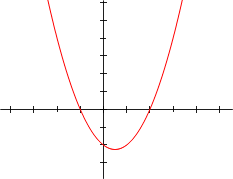# Nonlinear Regression: Simple Definition & Examples

Regression Analysis > Nonlinear Regression

## What is Nonlinear Regression?

Nonlinear regression uses nonlinear regression equations, which take the form:
Y = f(X,β) + ε
Where:

• X = a vector of p predictors,
• β = a vector of k parameters,
• f(-) = a known regression function,
• ε = an error term.

The formal definition is that if your regression equation looks like the one above, it’s nonlinear regression. However, this is actually a lot more difficult than it sounds. Take the following nonlinear regression equations:

These both meet the requirement of fitting the form Y = f(X,β) + ε, but that isn’t immediately obvious without some in-depth knowledge of algebra and regression analysis.

The good news is there is a much simpler, more intuitive definition of nonlinear regression:

If your model uses an equation in the form Y = a0 + b1X1, it’s a linear regression model. If not, it’s nonlinear.

It’s much easier to spot a linear regression equation, as it’s always going to take the form Y = a0 + b1X1*.

## Linear vs. Nonlinear RegressionLinear regression can, surprisingly, produce curves.Many people think that the difference between linear and nonlinear regression is that linear regression involves lines and nonlinear regression involves curves. This is partly true, and if you want a loose definition for the difference, you can probably stop right there. However, linear equations can sometimes produce curves.

In order to understand why, you need to take a look at the linear regression equation form.

Linear regression uses a linear equation in one basic form, Y = a +bx, where x is the explanatory variable and Y is the dependent variable:

Y = a0 + b1X1.

You can have multiple equations added together:
Y = a0 + b1X1 + b2X2 + b3X3
And you can even square a term to model a curve:
Y = a0 + b1X12.
Even though it’s modeling a curve, it’s still a linear regression equation because it’s in the form Y = a +bx.

A nonlinear regression equation can take on multiple forms.

It’s worth highlighting the intuitive definition again: If your equation looks like the examples above (i.e. it looks like Y = a +bx), it’s linear. If not, it’s nonlinear.

Note/caveat/disclaimer (AKA, there’s always an exception in statistics):* It’s true that if your model has an equation in the form Y = a +bx, then it’s linear. However, there are a few cases where a nonlinear equation can be transformed to mimic a linear equation. If this happens, the nonlinear equation is called “intrinsically linear.” For example, the nonlinear
Y = Β0X / (Β1 + X)
can be transformed with a little algebra to become intrinsically linear:
1/Y = 1/β0 + (β10)*1/X
= θ0 + θ1*1/X.

CITE THIS AS:
Stephanie Glen. "Nonlinear Regression: Simple Definition & Examples" From StatisticsHowTo.com: Elementary Statistics for the rest of us! https://www.statisticshowto.com/nonlinear-regression/
---------------------------------------------------------------------------Need help with a homework or test question? With Chegg Study, you can get step-by-step solutions to your questions from an expert in the field. Your first 30 minutes with a Chegg tutor is free!